# ShuffleNet-3D V2 Trained onVideo Datasets

Identify the main action in a video

Released in 2019, this family of nets consists of three-dimensional (3D) versions of the original ShuffleNet V2 architecture for video classification. The ShuffleNet V2 architecture utilizes a simple channel split operation that splits the input feature channel into two channels, reducing the costs of group convolutions. With the availability of large-scale video datasets such as Jester and Kinetics-600, these models achieve much better accuracies compared to their two-dimensional counterparts for video classification task.

Number of models: 8

## Training Set Information

• Kinetics-600 dataset, containing six hundred human action classes with at least six hundred video clips for each action. For each class, there are also 50 and one hundred validation and test videos respectively. Jester dataset, containing 148,092 gesture videos under 27 classes.

## Performance

• The models achieve the following accuracies on the original ImageNet validation set.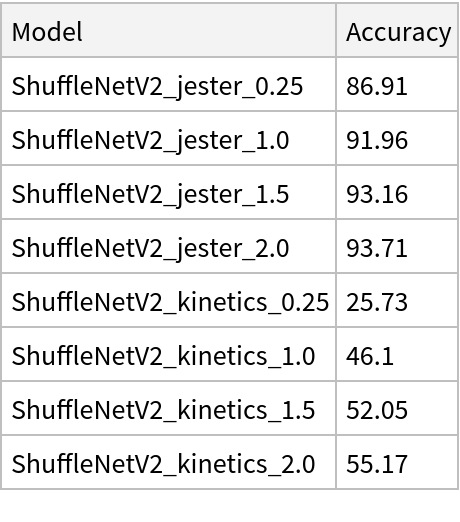## Examples

### Resource retrieval

Get the pre-trained net:

 In:=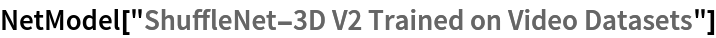Out=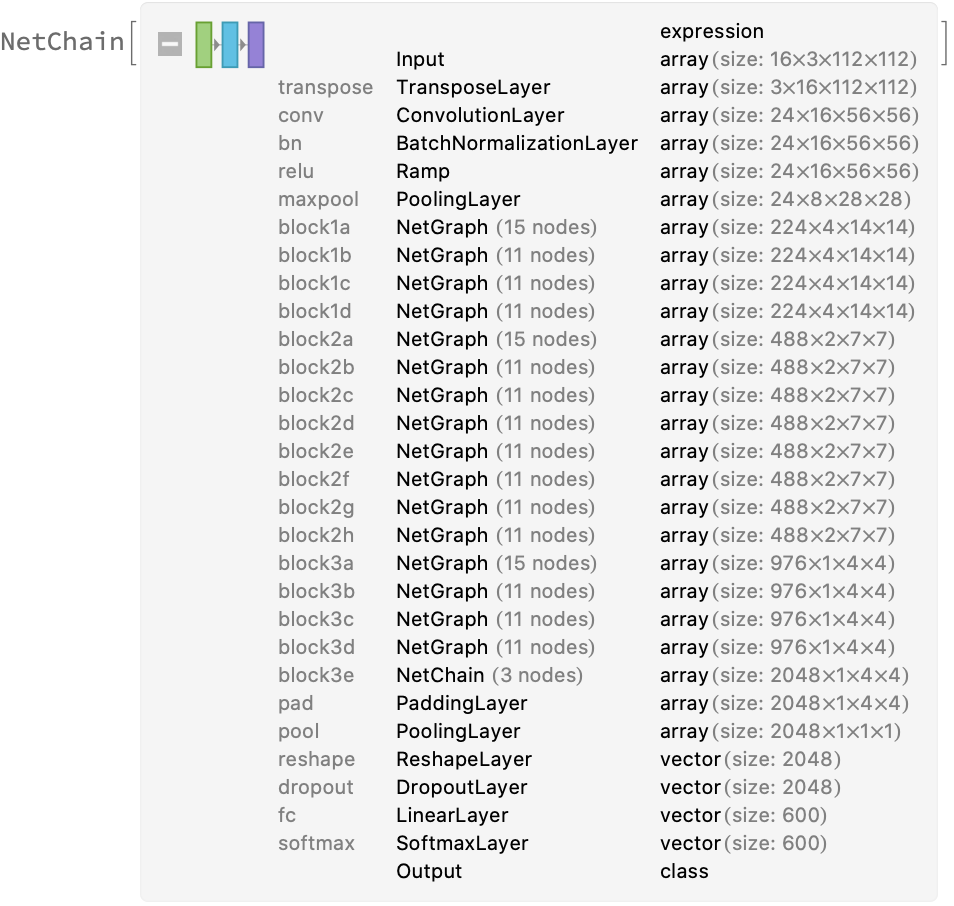### NetModel parameters

This model consists of a family of individual nets, each identified by a specific parameter combination. Inspect the available parameters:

 In:=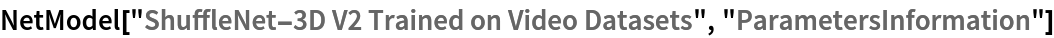Out=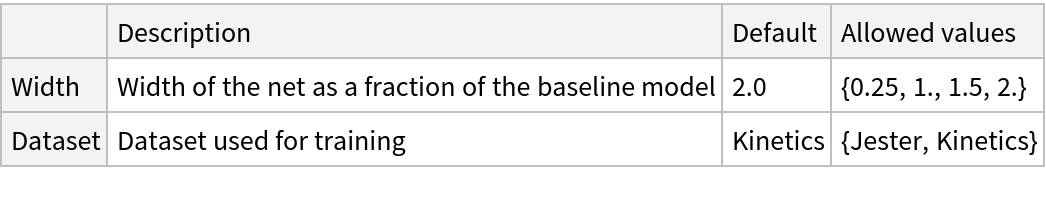Pick a non-default net by specifying the parameters:

 In:=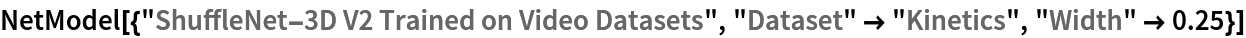Out=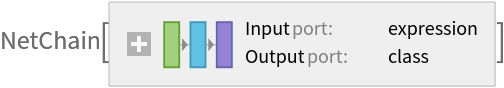Pick a non-default uninitialized net:

 In:=Out=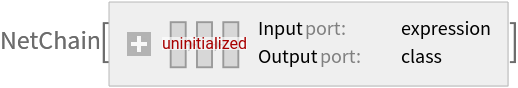### Basic usage

Identify the main action in a video:

 In:=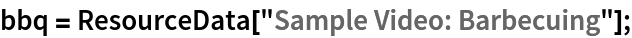In:=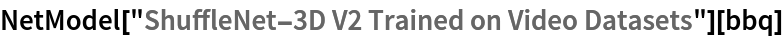Out=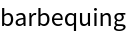Obtain the probabilities of the 10 most likely entities predicted by the net:

 In:=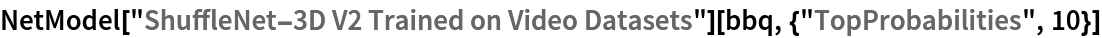Out=Obtain the list of names of all available classes:

 In:=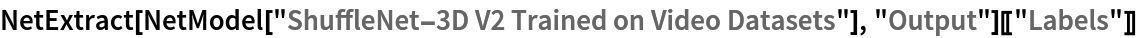Out=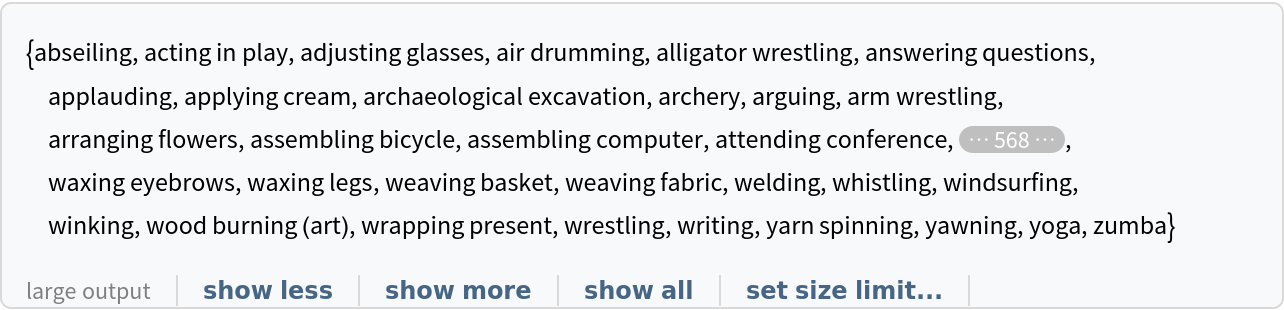### Network architecture

In addition to the channel shuffle operations introduced in ShuffleNet-3D V1, the ShuffleNet-3D V2 architecture reduces the costs using a simple operator called "channel split," which splits the input of c feature channels into two branches with c/2 channels. This reduces the costs of group convolutions and creates more "balanced" convolutions (with equal channel width). In addition, elementwise operations like ReLU and depthwise convolutions exist only in one branch. After convolution, the two branches are concatenated so that the channel numbers are the same:

 In:=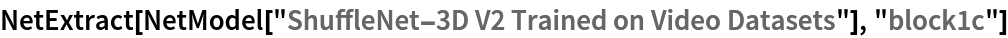Out=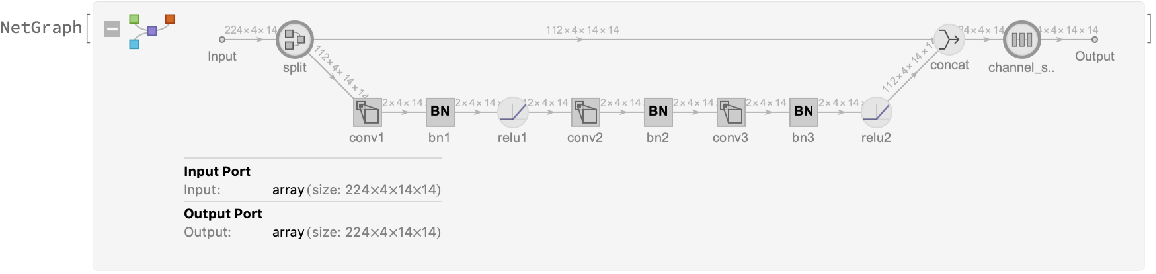For spatial down sampling the channel split operator is removed. Thus the number of channels on each branch is doubled:

 In:=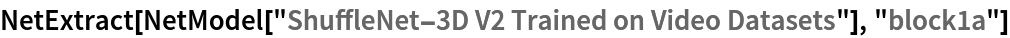Out=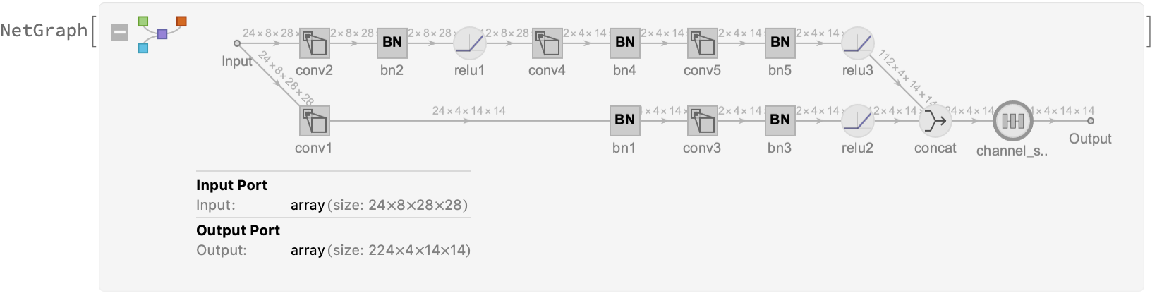### Feature extraction

Remove the last two layers of the trained net so that the net produces a vector representation of an image:

 In:=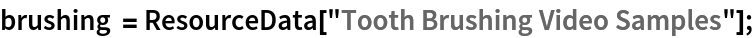In:=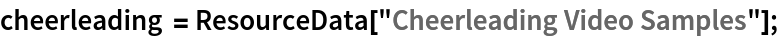In:=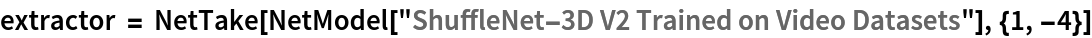Out=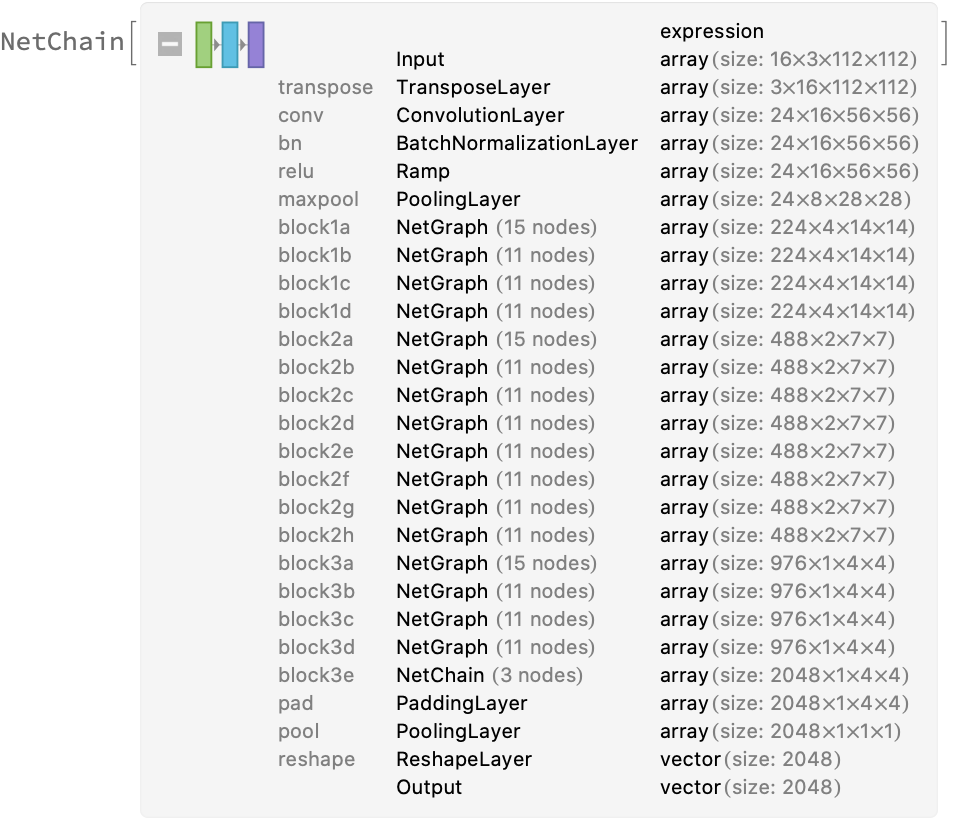Get a set of videos:

 In:=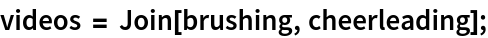Visualize the features of a set of videos:

 In:=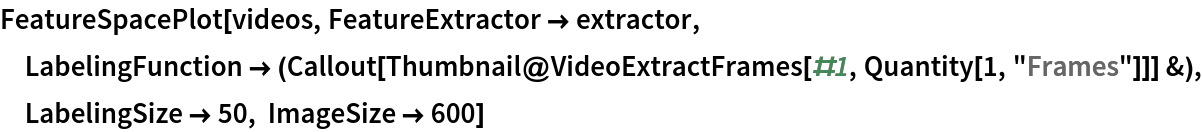Out=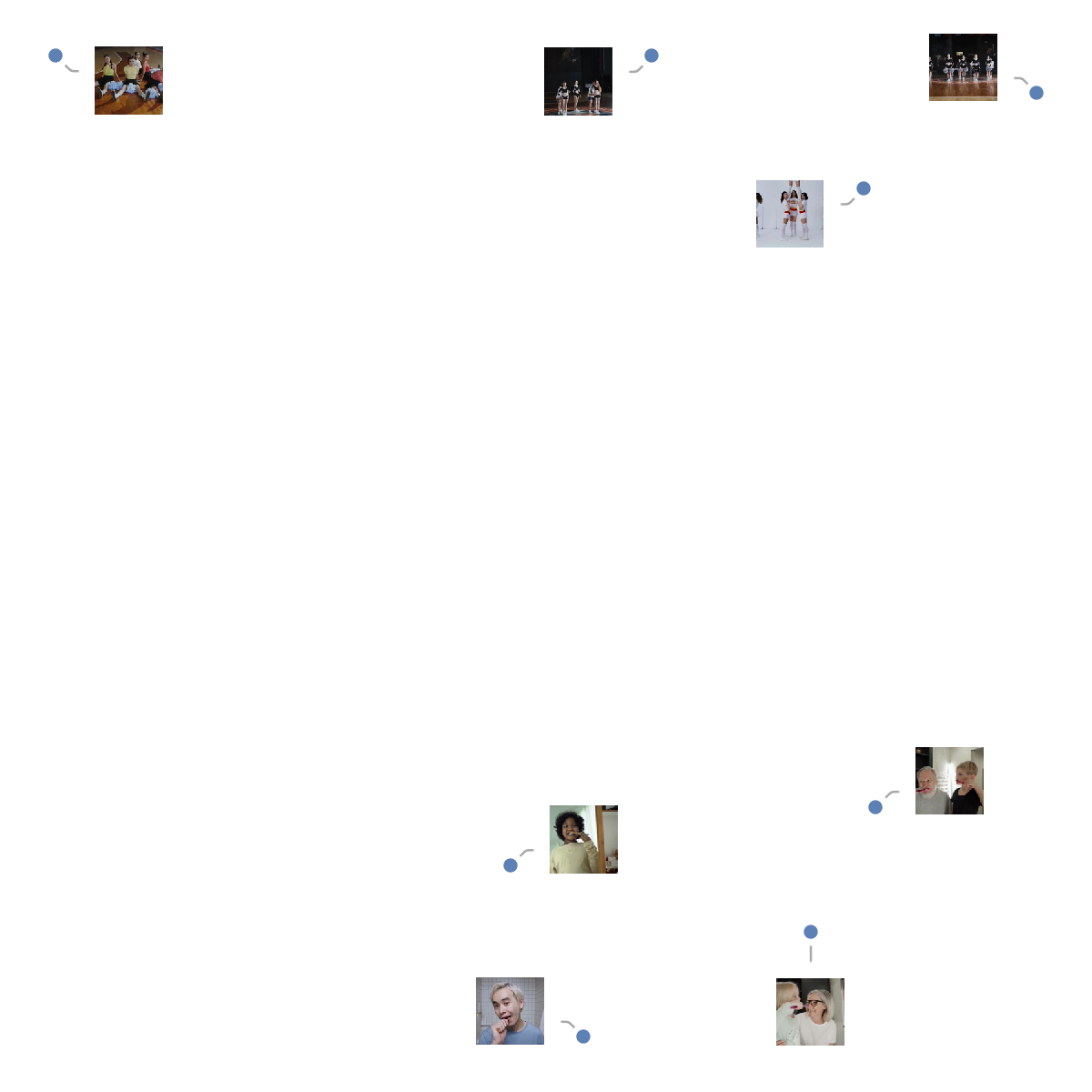### Transfer learning

Use the pre-trained model to build a classifier for telling apart images from two action classes not present in the dataset. Create a test set and a training set:

 In:=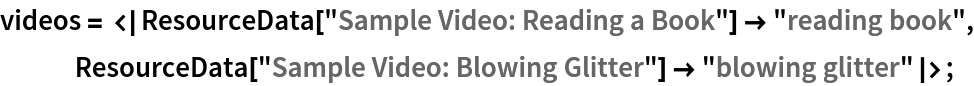In:=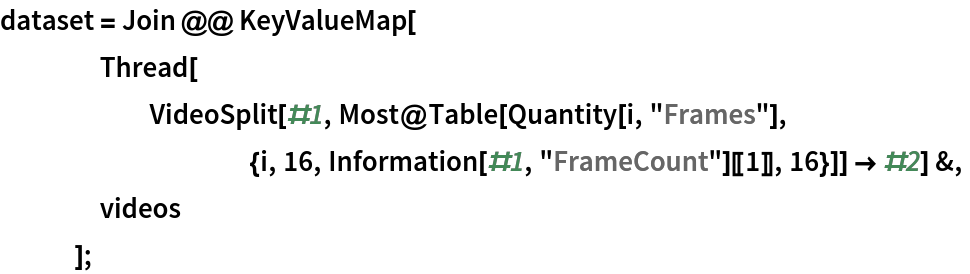In:=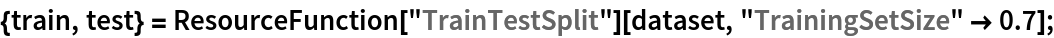Remove the linear layer from the pre-trained net:

 In:=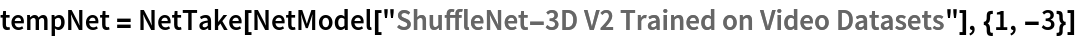Out=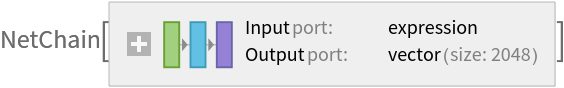Create a new net composed of the pre-trained net followed by a linear layer and a softmax layer:

 In:=Out=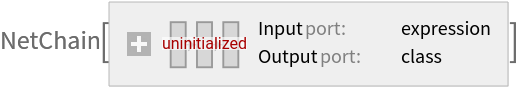Train on the dataset, freezing all the weights except for those in the "Linear" new layer (use TargetDevice -> "GPU" for training on a GPU):

 In:=Out=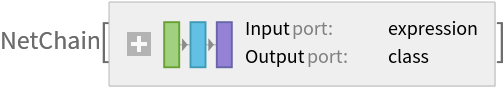Perfect accuracy is obtained on the test set:

 In:=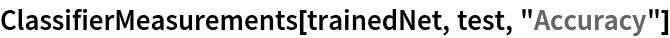Out=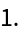### Net information

Inspect the number of parameters of all arrays in the net:

 In:=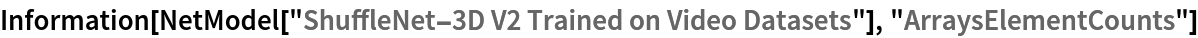Out=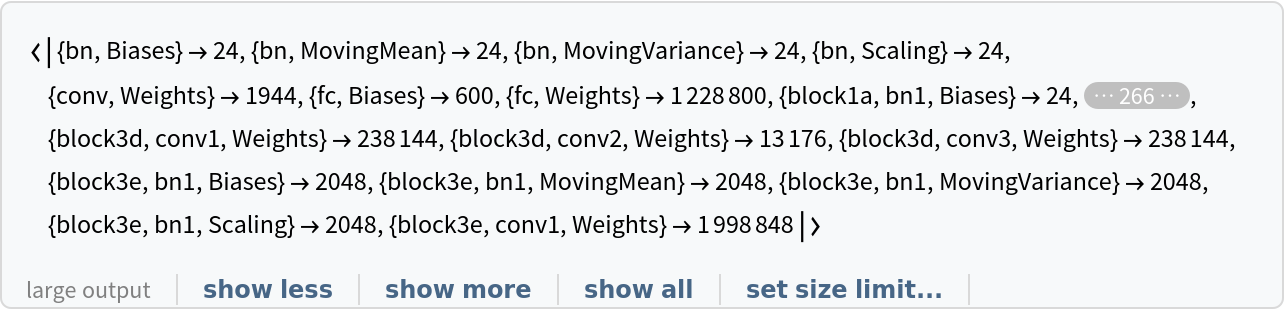Obtain the total number of parameters:

 In:=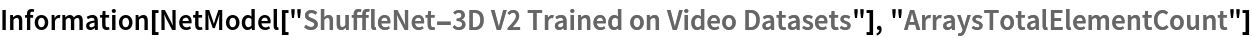Out=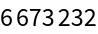Obtain the layer type counts:

 In:=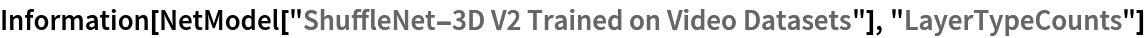Out=Display the summary graphic:

 In:=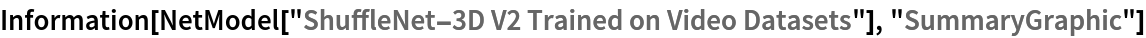Out=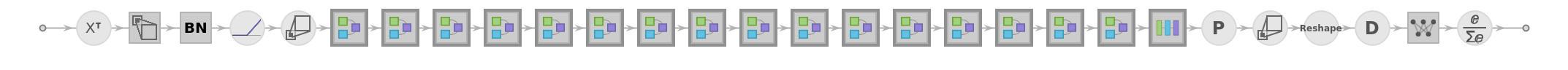### Export to ONNX

Export the net to the ONNX format:

 In:=Out=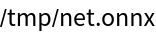Get the size of the ONNX file:

 In:=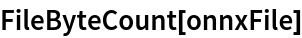Out=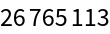Check some metadata of the ONNX model:

 In:=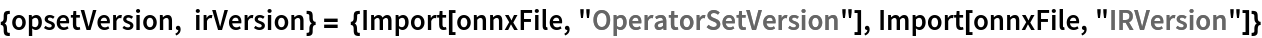Out=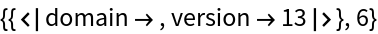Import the model back into the Wolfram Language. However, the NetEncoder and NetDecoder will be absent because they are not supported by ONNX:

 In:=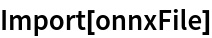Out=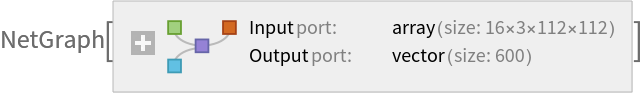## Reference

• O. Köpüklü, N. Kose, A. Gunduz, G. Rigoll, "Resource Efficient 3D Convolutional Neural Networks," arXiv:1904.02422 (2019)